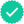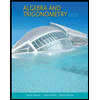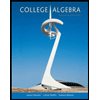#### Related questions#### Concept explainers

Question

A bag contains total 30 balls out of which 9 are red, 12 are white and 9 are blue. Two balls are drawn in succession without replacement.
What is the probability that first is red and second is white?

Expert SolutionStep 1

Let us first find probability that the first ball is red. For that we will need the following.

Since, there are total 30 balls out which 9 are red, we have:

Step 2

Probability (P1), that the first ball is red, is now given as:

Step 3

Let us now find probability that the second ball is white. For that we will need the following.

Since, one ball is already drawn; total balls left, now, are 29 out of which 12 are white. We have:Trending nowThis is a popular solution!Step by stepSolved in 5 steps with 5 imagesKnowledge BoosterRecommended textbooks for you
•Algebra and Trigonometry (MindTap Course List)
Algebra
ISBN:9781305071742
Author:James Stewart, Lothar Redlin, Saleem Watson
Publisher:Cengage LearningCollege Algebra
Algebra
ISBN:9781305115545
Author:James Stewart, Lothar Redlin, Saleem Watson
Publisher:Cengage Learning
•Algebra and Trigonometry (MindTap Course List)
Algebra
ISBN:9781305071742
Author:James Stewart, Lothar Redlin, Saleem Watson
Publisher:Cengage LearningCollege Algebra
Algebra
ISBN:9781305115545
Author:James Stewart, Lothar Redlin, Saleem Watson
Publisher:Cengage Learning